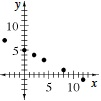### Home > MC1 > Chapter 9 > Lesson 9.1.1 > Problem9-10

9-10.

Copy the table below on your own graph paper.

 x y $4$ $0$ $–4$ $2$ $12$ $8$ $3$ $5$ $7$ $4$ $–1$ $1$
1. Draw axes and plot these points.2. Add three new points to your graph and your table that satisfy the same rule as the points given.

What pattern does the graph follow? Can you find other points that follow the same pattern?